# Ni + PbO2+ 4H+ Pb2+ + Ni2++ 21,0 In the above redox reaction, use oxidation numbers...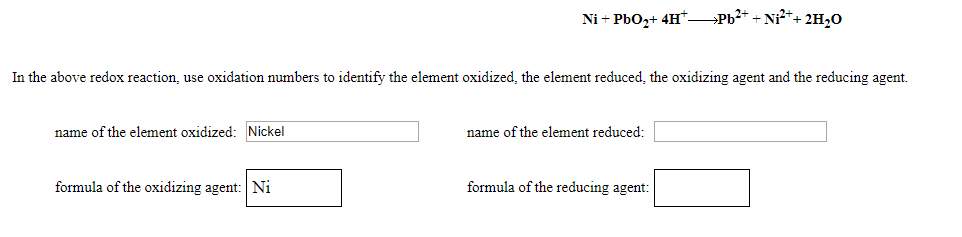Ni + PbO2+ 4H+ Pb2+ + Ni2++ 21,0 In the above redox reaction, use oxidation numbers to identify the element oxidized the element reduced the oxidizing agent and the reducing agent. name of the element oxidized: Nickel name of the element reduced: formula of the oxidizing agent: Ni formula of the reducing agent:

Name of the element oxidized : Nickel (Ni)

Name of the element reduced : Lead (Pb)

​​​​​formula of the oxidizing agent : PbO2

formula of the reducing agent : Ni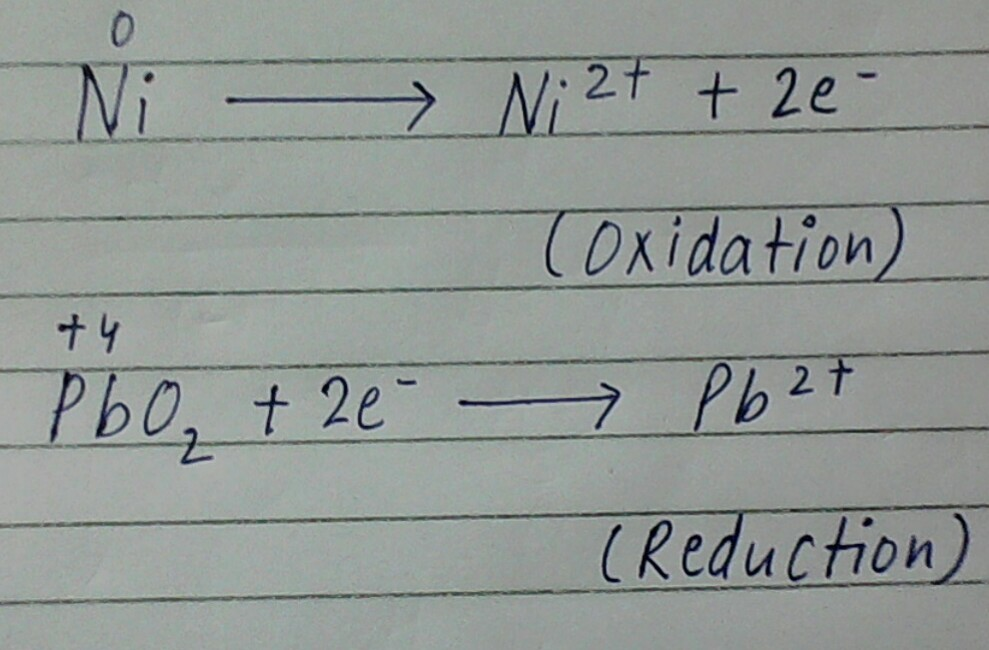---------------------------------------------------------------------------------

Electron losing species is called reducing agent. While, electron accepting species is called oxidizing agent.

#### Earn Coin

Coins can be redeemed for fabulous gifts.

Similar Homework Help Questions
• ### Ni(OH)2 + NO3- _______> NiO2 + NO2- + H2O In the above redox reaction, use oxidation numbers to identify the...

Ni(OH)2 + NO3- _______> NiO2 + NO2- + H2O In the above redox reaction, use oxidation numbers to identify the element oxidized, the element reduced, the oxidizing agent and the reducing agent. name of the element oxidized:    name of the element reduced: formula of the oxidizing agent: formula of the reducing agent:

• ### Cd2+ + Ni2++ 2H2O-Cd+NiO2+ 4H* In the above redox reaction, use oxidation numbers to identify the...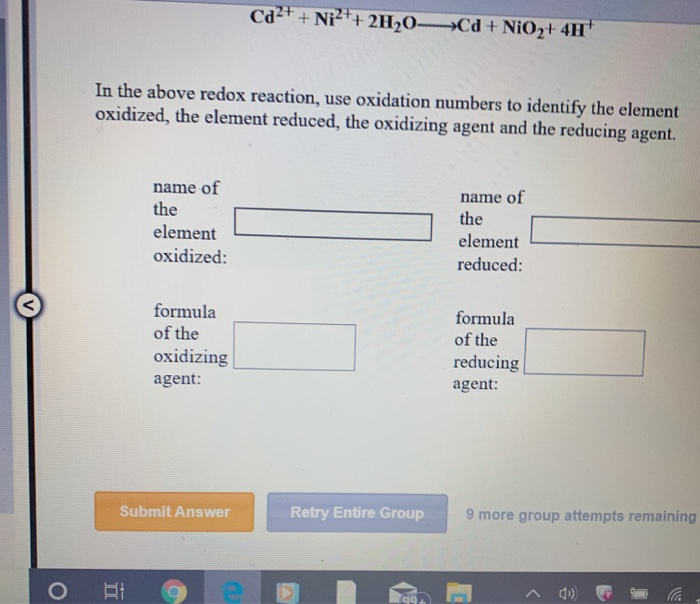Cd2+ + Ni2++ 2H2O-Cd+NiO2+ 4H* In the above redox reaction, use oxidation numbers to identify the element oxidized, the element reduced, the oxidizing agent and the reducing agent. name of the element oxidized: name of the element reduced: formula of the oxidizing agent: formula of the reducing agent: Submit Answer Retry Entire Group 9 more group attempts remaining о вое БТ Identify the species oxidized, the species reduced, the oxidizing agent a reducing agent in the following electron transfer reaction....

• ### 10Cr3+ + 6IO3-+ 22H2O10CrO42- + 3I2+ 44H+ In the above redox reaction, use oxidation numbers to...

10Cr3+ + 6IO3-+ 22H2O10CrO42- + 3I2+ 44H+ In the above redox reaction, use oxidation numbers to identify the element oxidized, the element reduced, the oxidizing agent and the reducing agent. name of the element oxidized: name of the element reduced: formula of the oxidizing agent: formula of the reducing agent:

• ### Can somebody help me on this? please In the above redox reaction, use oxidation numbers to...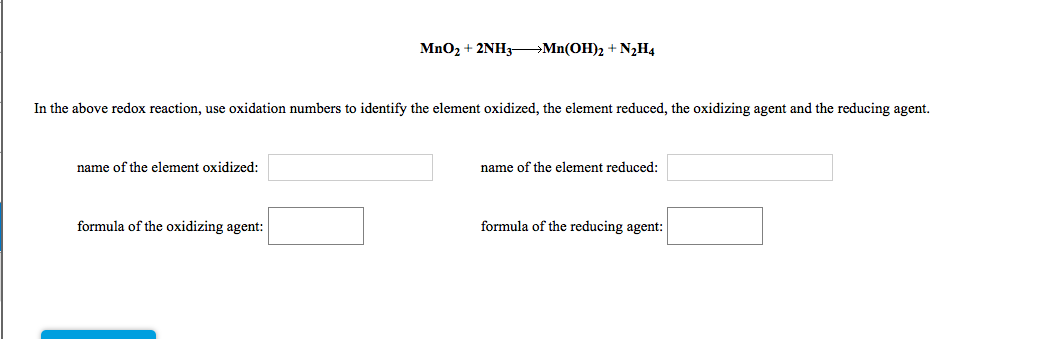Can somebody help me on this? please In the above redox reaction, use oxidation numbers to identify the element oxidized, the element reduced, the oxidizing agent and the reducing agent. name of the element oxidized: name of the element reduced: formula of the oxidizing agent: formula of the reducing agent:

• ### 2NO 3CIO4H202HNO3+ 3CI03 In the above redox reaction, use oxidation numbers to identify the element oxidized,...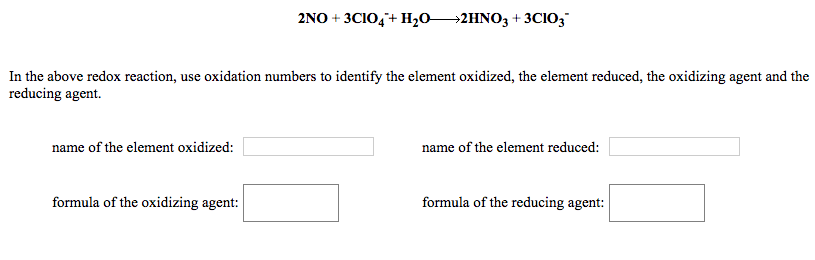2NO 3CIO4H202HNO3+ 3CI03 In the above redox reaction, use oxidation numbers to identify the element oxidized, the element reduced, the oxidizing agent and the reducing agent. name of the element reduced name of the element oxidized: formula of the oxidizing agent formula of the reducing agent:

• ### 2BrO35NO2 H20 >Br2+ 5NO3+ 20H In the above redox reaction, use oxidation numbers to identify the...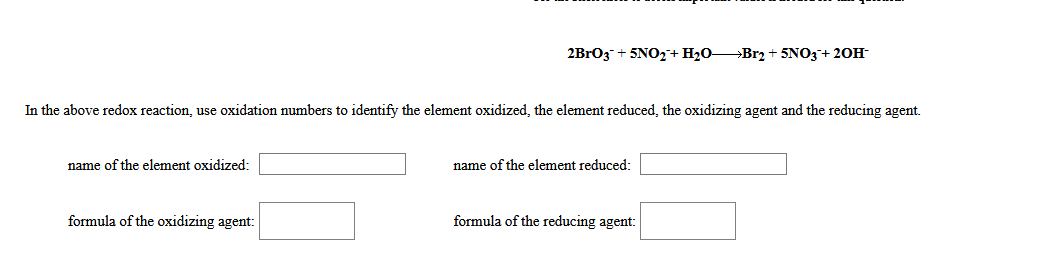2BrO35NO2 H20 >Br2+ 5NO3+ 20H In the above redox reaction, use oxidation numbers to identify the element oxidized, the element reduced, the oxidizing agent and the reducing agent. name of the element oxidized: name of the element reduced: formula of the oxidizing agent formula of the reducing agent

• ### 5Zn2+ + Clz+ 6H20— 5Zn +2010; +12H In the above redox reaction, use oxidation numbers to...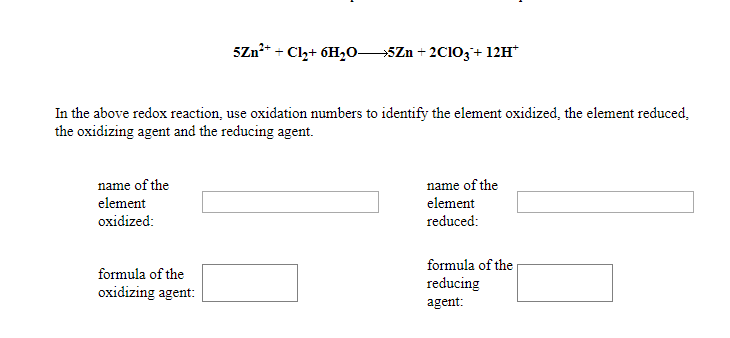5Zn2+ + Clz+ 6H20— 5Zn +2010; +12H In the above redox reaction, use oxidation numbers to identify the element oxidized, the element reduced. the oxidizing agent and the reducing agent. name of the element oxidized: name of the element reduced: formula of the oxidizing agent: formula of the reducing agent:

• ### 6HCIO + 2NO3Cl2 + 2NO3 + 2H+ 2H20 In the above redox reaction, use oxidation numbers to identify the element oxidiz...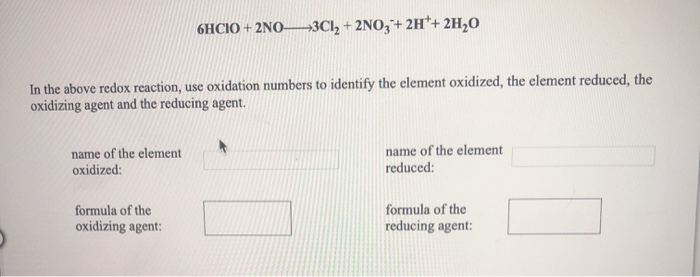6HCIO + 2NO3Cl2 + 2NO3 + 2H+ 2H20 In the above redox reaction, use oxidation numbers to identify the element oxidized, the element reduced, the oxidizing agent and the reducing agent. name of the element oxidized: name of the element reduced: formula of the oxidizing agent: formula of the reducing agent:

• ### 2Mn2+ + 5H3AsO45HAsO2 + 2MnO4-+ 6H++ 2H2O In the above redox reaction, use oxidation numbers to...2Mn2+ + 5H3AsO45HAsO2 + 2MnO4-+ 6H++ 2H2O In the above redox reaction, use oxidation numbers to identify the element oxidized, the element reduced, the oxidizing agent and the reducing agent. 2Cl2 + 5Sb2O5+ 6H+10SbO+ + 4ClO3-+ 3H2O In the above redox reaction, use oxidation numbers to identify the element oxidized, the element reduced, the oxidizing agent and the reducing agent. 2MnO4- + 3N2H4+ 4H2O6NH2OH + 2MnO2+ 2OH- In the above redox reaction, use oxidation numbers to identify the element oxidized,...

• ### Si + 2MnO2+ H2O+ 2OH-2Mn(OH)2 + SiO32- In the above redox reaction, use oxidation numbers to...

Si + 2MnO2+ H2O+ 2OH-2Mn(OH)2 + SiO32- In the above redox reaction, use oxidation numbers to identify the element oxidized, the element reduced, the oxidizing agent and the reducing agent. name of the element oxidized: name of the element reduced: formula of the oxidizing agent: formula of the reducing agent: